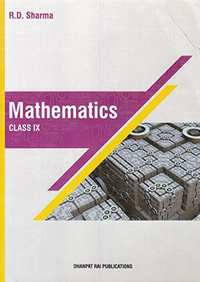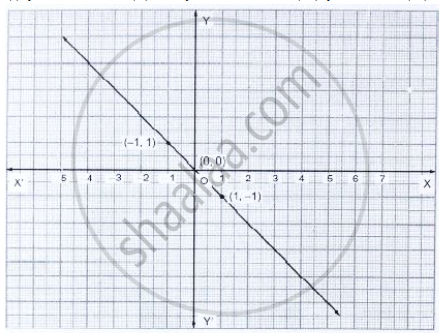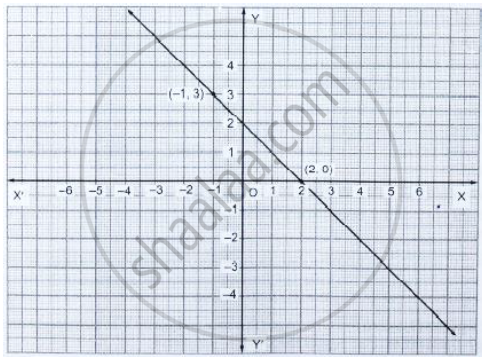Share

# RD Sharma solutions for Mathematics for Class 9 chapter 7 - Linear Equations in Two Variables [Latest edition]

Course
Textbook page

#### Chapters## Chapter 7: Linear Equations in Two Variables

#### RD Sharma solutions for Mathematics for Class 9 Chapter 7 Linear Equations in Two Variables

Q 1.1

Express the following linear equations in the form ax + by + c = 0 and indicate the values of
a, b and c in each case :

-2x + 3y = 12

Q 1.2

Express the following linear equations in the form ax + by + c = 0 and indicate the values of
a, b and c in each case:

x - y/2- 5 = 0

Q 1.3

Express the following linear equations in the form ax + by + c = 0 and indicate the values of
a, b and c in each case:

2x + 3y = 9.35

Q 1.4

Express the following linear equations in the form ax + by + c = 0 and indicate the values of
a, b and c in each case:

3x = -7y

Q 1.5

Express the following linear equations in the form ax + by + c = 0 and indicate the values of
a, b and c in each case:

2x + 3 = 0

Q 1.6

Express the following linear equations in the form ax + by + c = 0 and indicate the values of
a, b and c in each case:

y – 5 = 0

Q 1.7

Express the following linear equations in the form ax + by + c = 0 and indicate the values of
a, b and c in each case:

4 = 3x

Q 1.8

Express the following linear equations in the form ax + by + c = 0 and indicate the values of
a, b and c in each case:

y = x / 2

Q 2.1

Write  the following as an equation in two variable :

2x = −3

Q 2.2

Write the following as an equation in two variable :

y = 3

Q 2.3

Write the following as an equation in two variable :

5x = 7/2

Q 2.4

Write the following as an equation in two variable :

y = 3/2 x

Q 3

The cost of ball pen is Rs. 5 less than half of the cost of fountain pen. Write this statement as
a linear equation in two variables.

#### RD Sharma solutions for Mathematics for Class 9 Chapter 7 Linear Equations in Two Variables

Q 1.1

Write two solutions for the following equation :

3x + 4y = 7

Q 1.2

Write two solutions of the following equation :

x = 6y

Q 1.3

Write two solutions for the following equation :

x + 𝜋y = 4

Q 1.4

Write two solutions for the following equation :

2/3 x - y = 4

Q 2.1

Write two solutions of the form x = 0, y = a and x = b, y = 0 for the following equation :

5x – 2y = 10

Q 2.2

Write two solutions of the form x = 0, y = a and x = b, y = 0 for the following equation :

−4x + 3y = 12

Q 2.3

Write two solutions of the form x = 0, y = a and x = b, y = 0 for the following equation :

2𝑥 + 3𝑦 = 24

Q 3

Check which of the following are solutions of the equation 2x – y = 6 and which are not :

(1) (3, 0)    (2) (0, 6)    (3) (2, −2)     (4) (√3, 0 )    (5) (1/2,-5)

Q 4

If x = −1, y = 2 is a solution of the equation 3x + 4y = k, find the value of k.

Q 5

Find the value of 𝜆, if x = −𝜆 and y =5/2
is a solution of the equation x + 4y – 7 = 0.

Q 6

If x = 2𝛼 + 1 and y = 𝛼 – 1 is a solution of the equation 2x−3y + 5 = 0, find the value of 𝛼.

Q 7

If x = 1 and y = 6 is a solution of the equation 8x – ay + a2 = 0, find the value of a.

#### RD Sharma solutions for Mathematics for Class 9 Chapter 7 Linear Equations in Two Variables

Q 1.1

Draw the graph of the following linear equations in two variables:- x + y = 4

Q 1.2

Draw the graph of the following linear equations in two variables:- x – y = 2

Q 1.3

Draw the graph of the following linear equation in two variable : –x + y = 6

Q 1.4

Draw the graph of  the following linear equation in two variable : y = 2x

Q 1.5

Draw the graph of the following linear equation in two variable : 3x + 5y = 15

Q 1.6

Draw the graph of the following linear equation in two variable :  x / 2 - y/ 3 = 2

Q 1.7

Draw the graph of the following linear equation in two variable :  (x-2)/3 = y - 3

Q 1.8

Draw the graph of the following linear equations in two variable : 2𝑦 = −𝑥 + 1

Q 2

Give the equations of two lines passing through (3, 12). How many more such lines are there,
and why?

Q 3

A three-wheeler scooter charges Rs 15 for first kilometer and Rs 8 each for every subsequent
kilometer. For a distance of x km, an amount of Rs y is paid. Write the linear equation
representing the above information.

Q 4

A lending library has a fixed charge for the first three days and an additional charge for each
day thereafter. Aarushi paid Rs 27 for a book kept for seven days. If fixed charges are Rs 𝑥
and per day charges are Rs y. Write the linear equation representing the above information.

Q 5

A number is 27 more than the number obtained by reversing its digits. If its unit’s and ten’s
digit are x and y respectively, write the linear equation representing the above statement.

Q 6

The sum of a two digit number and the number obtained by reversing the order of its digits
is 121. If units and ten’s digit of the number are x and y respectively then write the linear
equation representing the above statement.

Q 7

Plot the points (3, 5) and (− 1, 3) on a graph paper and verify that the straight line passing
through these points also passes through the point (1, 4).

Q 8

From the choices given below, choose the equation whose graph is given in Fig. below.
(i) y = x    (ii) x + y = 0    (iii) y = 2x   (iv) 2 + 3y = 7x[Hint: Clearly, (-1, 1) and (1, -1) satisfy the equation x + y = 0]

Q 9

From the choices given below, choose the equation whose graph is given in fig. below.
(i)  y = x + 2   (ii)  y = x – 2   (iii) y = −x + 2   (iv) x + 2y = 6[Hint: Clearly, (2, 0) and (−1, 3) satisfy the equation y = −x + 2]

Q 10

If the point (2, -2) lies on the graph of the linear equation 5x + ky = 4, find the value of k.

Q 11

Draw the graph of the equation 2x + 3y = 12. From the graph, find the coordinates of the
point: (i) whose y-coordinates is 3. (ii) whose x-coordinate is −3.

Q 12.1

Draw the graph of the equation given below. Also, find the coordinates of the point
where the graph cuts the coordinate axes :  6x − 3y = 12

Q 12.2

Draw the graph of the equation given below. Also, find the coordinates of the point
where the graph cuts the coordinate axes :  −x + 4y = 8

Q 12.3

Draw the graph of the equation given below. Also, find the coordinates of the point
where the graph cuts the coordinate axes :  2x + y = 6

Q 12.4

Draw the graph of the equation given below. Also, find the coordinate of the points
where the graph cuts the coordinate axes : 3x + 2y + 6 = 0

Q 13

Draw the graph of the equation 2x + y = 6. Shade the region bounded by the graph and the
coordinate axes. Also, find the area of the shaded region.

Q 14

Draw the graph of the equatio  x / y + y /4 = 1 Also, find the area of the triangle formed by the
line and the co-ordinates axes.

Q 15

Draw the graph of y = | x |.

Q 16

Draw the graph of y = | x | + 2.

Q 17

Draw the graphs of the following linear equations on the same graph paper: 2x + 3y = 12, x
– y = 1.
Find the coordinates of the vertices of the triangle formed by the two straight lines and the
y-axis. Also, find the area of the triangle.

Q 18

Draw the graphs of the linear equations 4x − 3y + 4 = 0 and 4x + 3y − 20 = 0. Find the area
bounded by these lines and x-axis.

Q 19

The path of a train A is given by the equation 3x + 4y − 12 = 0 and the path of another train
B is given by the equation 6x + 8y − 48 = 0. Represent this situation graphically.

Q 20

Ravish tells his daughter Aarushi, “Seven years ago, I was seven times as old as you were
then. Also, three years from now, I shall be three times as old as you will be”. If present ages
of Aarushi and Ravish are x and y years respectively, represent this situation algebraically as
well as graphically.

Q 21

Aarushi was driving a car with uniform speed of 60 km/h. Draw distance-time graph. From
the graph, find the distance travelled by Aarushi in

(1)   2 1/2  Hours      (2)  1/2  Hour

#### RD Sharma solutions for Mathematics for Class 9 Chapter 7 Linear Equations in Two Variables

Q 1.1
Give the geometric representation of the following equation
(a) on the number line   (b) on the Cartesian plane :  x = 2
Q 1.2

Give the geometric representation of the following equation
(a) on the number line    (b) on the Cartesian plane  :  y + 3 = 0

Q 1.3

Give the geometric representation of the following equation
(a) on the number line   (b) on the Cartesian plane :   y = 3

Q 1.4

Give the geometric representation  of the following equation
(a) on the number line    (b) on the Cartesian plane :   2x + 9 = 0

Q 1.5

Give the geometric representation of the following equation
(a) on the number line     (b) on the Cartesian plane :   3x – 5 = 0

Q 2.1

Give the geometrical representation of 2x + 13 = 0 as an equation in one variable .

Q 2.2

Give the geometrical representation of 2x + 13 = 0 as an equation in two variables .

Q 3.1

Solve the equation 3x + 2 = x − 8, and represent the solution on the number line .

Q 3.2

Solve the equation 3x + 2 = x − 8, and represent the solution on the
Cartesian plane .

Q 4.1

Write the equation of the line that is parallel to x-axis and passing through the point  (0, 3) .

Q 4.2

Write the equation of the line that is parallel to x-axis and passing through the point   (0, -4) .

Q 4.3

Write the equation of the line that is parallel to x-axis and passing through the point  (2, -5) .

Q 4.4

Write the equation of the line that is parallel to x-axis and passing through the point  (3, 4) .

Q 5.1

Write the equation of the line that is parallel to y-axis and passing through the point  (4, 0) .

Q 5.2

Write the equation of the line that is parallel to y-axis and passing through the point  (−2, 0 ) .

Q 5.3

Write the equation of the line that is parallel to y-axis and passing through the point (3, 5) .

Q 5.4

Write the equation of the line that is parallel to y-axis and passing through the point  (− 4, −3) .

## Chapter 7: Linear Equations in Two Variables## RD Sharma solutions for Mathematics for Class 9 chapter 7 - Linear Equations in Two Variables

RD Sharma solutions for Mathematics for Class 9 chapter 7 (Linear Equations in Two Variables) include all questions with solution and detail explanation. This will clear students doubts about any question and improve application skills while preparing for board exams. The detailed, step-by-step solutions will help you understand the concepts better and clear your confusions, if any. Shaalaa.com has the CBSE Mathematics for Class 9 solutions in a manner that help students grasp basic concepts better and faster.

Further, we at Shaalaa.com provide such solutions so that students can prepare for written exams. RD Sharma textbook solutions can be a core help for self-study and acts as a perfect self-help guidance for students.

Concepts covered in Mathematics for Class 9 chapter 7 Linear Equations in Two Variables are Introduction of Linear Equations, Solution of a Linear Equation, Graph of a Linear Equation in Two Variables, Concept of Linear Equations in Two Variables, Equations of Lines Parallel to the X-axis and Y-axis.

Using RD Sharma Class 9 solutions Linear Equations in Two Variables exercise by students are an easy way to prepare for the exams, as they involve solutions arranged chapter-wise also page wise. The questions involved in RD Sharma Solutions are important questions that can be asked in the final exam. Maximum students of CBSE Class 9 prefer RD Sharma Textbook Solutions to score more in exam.

Get the free view of chapter 7 Linear Equations in Two Variables Class 9 extra questions for Mathematics for Class 9 and can use Shaalaa.com to keep it handy for your exam preparation

S